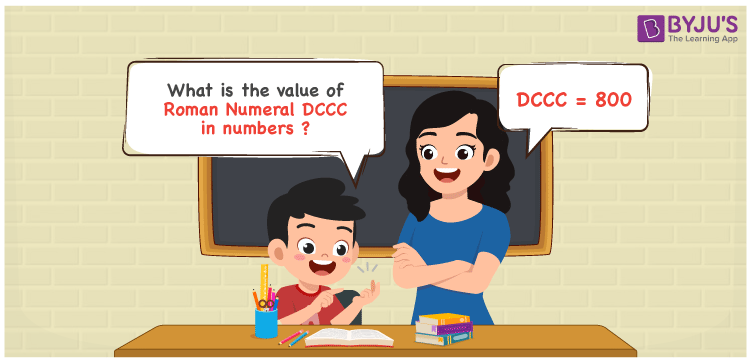# 800 in Roman Numerals

DCCC is 800 in Roman numerals. We’ll write 800 in expanded form, i.e. 800 = 500 + 100 + 100, to convert 800 to Roman Numerals. We get 800 = D + C + C + C = DCCC by substituting the converted numbers with their appropriate roman numerals. In this article, we’ll show you how to convert 800 to Roman numerals accurately.

 Number Roman Numeral 800 DCCC## How to Write 800 in Roman Numerals?

In order to write 800 in Roman Numerals, it has to be written in expanded form, i.e.

800 = 500 + 100 + 100 + 100

800 = D + C + C + C

800 = DCCC

## Video Lesson on Roman Numerals## Frequently Asked Questions on 800 in Roman Numerals

Q1

### Write 800 in Roman Numerals.

In Roman Numerals, 800 is written as DCCC.
Q2

### Why is 800 in Roman Numerals Written as DCCC?

We know that in roman numerals, we write 500 as D, and 100 as C.
Therefore, 800 in roman numerals is written as 800 = DCCC.
Q3

### How to convert 800 into Roman Numerals?

The conversion of 800 into Roman Numerals includes breaking the number according to its place value.
Hundreds = 800 = DCCC
Number = DCCC